# Linear regression

Linear regression, also called ‘Line of best fit’, is a statistical tool that models the relationship between several variables. The linear regression creates a median line that passes as close as possible to the average of all the points. It is especially useful to understand the correlation between two business factors, e.g. how much one variable impacts the other one. It is quite easy to model in an Excel spreadsheet. With careful analysis and interpretation, it can be a great input in elaborating strategic scenarios.

## What to use it for.

What it does Example
Checks if a correlation exists between two variables Link between school and home performance of the BU?
Measures relevance of the explanatory variable What % of performance is explained by highlighting?
Measure the impact of leverage on a result What additional TO if 10% of additional development?
Set a goal for improving underperforming entities What is the unit cost objective given the size of the BU?
Predict future developments or goals – in line with inputs Help set sales target consistent with investment

## How it works.

The linear regression line y = ax + b is the one that passes closest points Mi according to the least squares method. The right (model) minimizes the sum :
Σ (right model – real point)²

Covariance = average product of deviations from the mean
V (X) = variance = mean square deviations from the mean
σ (X) = standard deviation = square root of the variance

Measuring the quality of the regression:
R = correlation coefficient (range -1 to 1) = cov (X,Y) / [ σ (X) . σ (Y) ]
R² = coefficient of determination (between 0 and 1) = [ cov (X,Y) ]² / [ V (X) . V (Y) ]
If the variables are independent, the covariance is low, and low R ²
If the variables are related, R is close to 1 or -1, R ² close to 1, the points are close to the regression line.

WARNING: graphic validation is mandatory!

## How to use it.

1. Select variables and data sets
2. View (essential to interpret)
3. Graphically identify and eliminate parasites items
4. Calculate the regression and R²

## Related Microsoft Excel formulas.

SLOPE () calculates the slope of the linear regression line
CORREL () calculates R
RSQ () calculates R²
FORECAST () gives a value on the regression line along a linear trend

## Illustrations.

Example 1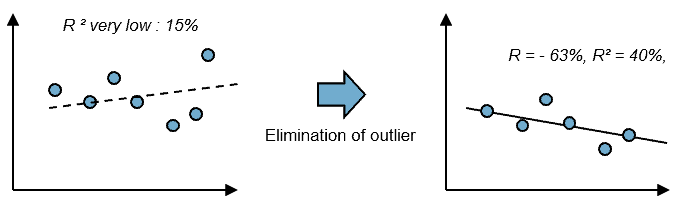In this first example, the R² is really low. However you can see that there is one point that stand out as an outlier. Eliminating this outlier point makes you to find a brand new correlation between all the points that is of much better quality (40% vs. 15%). This underlines the importance of the third point of how to use it: don’t forget to eliminate outlier items where appropriate. When doing this it is important to question whether the outlier is a genuine anomaly or whether it is the product of structural factors that you need to drill down into in greater depth.

Example 2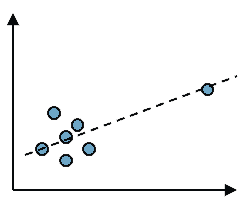This example again underlines the importance of eliminating outlier items. You see on this example how a single outlier point on the right lets you falsely believe that a correlation can be found between all these points. Remove that point and you soon realize that there clearly is no correlation between this set of dots. This also demonstrates the importance of visual representation and interpretation before you start any calculation. It helps you do a quick intuitive check into problems that any calculation is likely to encounter.

Example 3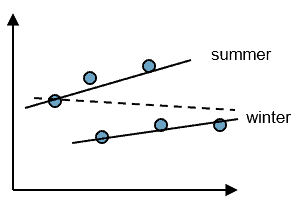Another example to show the importance of visalizing the data before diving into statistics. In this case, your data suggests no correlation. With a closer look you see that there are in fact two sets of data that are each highly correlated within their own group.

Example 4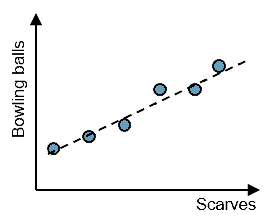When you find a high correlation between a set of points, take perspective before you interprete your data. Is there really a high correlation between bowling balls and scarves?

Example 5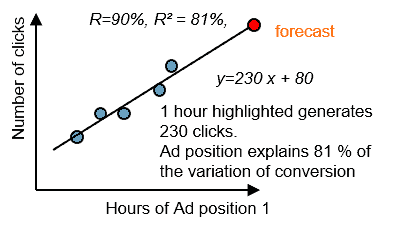This example shows that finding a good correlation between variables allows you to forecast business results. By finding the equation of your regression line, you are able to apply the formula to another set of data. Microsoft Excel will help you greatly in the process as you can ask the program to show you the equation on the graph. you can also use the forecast formula to calculate estimates directly from your initial set of data.

Tags:
1.